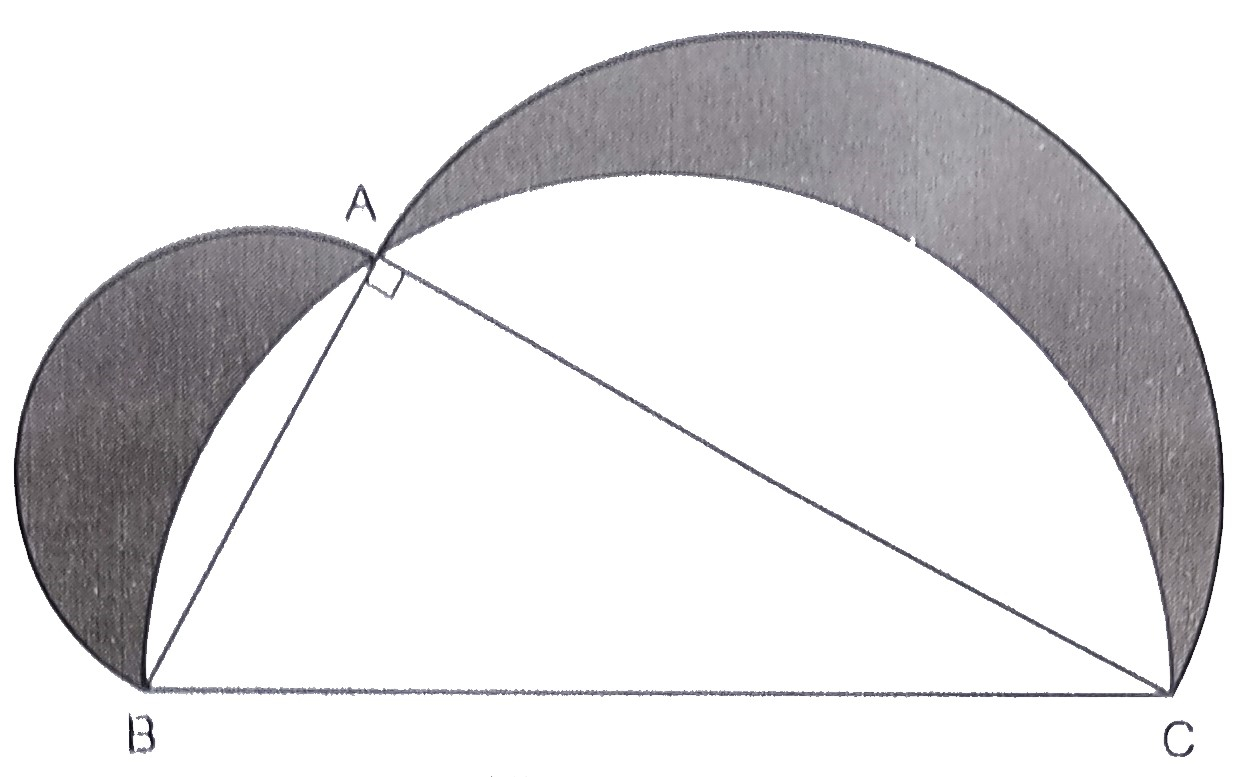"
">

# In the below figure, $A B C$ is a right angled triangle in which $\angle A=90^{\circ}, A B=21 \mathrm{~cm}$ and $A C=28 \mathrm{~cm} .$ Semi-circles are described on $A B, B C$ and $A C$ as diameters. Find the area of the shaded region."

Given:

$A B C$ is a right angled triangle in which $\angle A=90^{\circ}, A B=21 \mathrm{~cm}$ and $A C=28 \mathrm{~cm} .$ Semi-circles are described on $A B, B C$ and $A C$ as diameters.

To do:

We have to find the area of the shaded region.

Solution:

Semicircles are drawn on $BC$ and $AC$ as diameters.

In right triangle $ABC$, by Pythagoras theorem,

$BC^2 = AB^2+AC^2$

$= 21^2+ 28^2$

$= 441 + 784$

$= 1225$

$= (35)^2$

$\Rightarrow BC = 35\ cm$

Radius of the largest semicircle $R = \frac{35}{2}\ cm$

Radius of semicircle on $AB =\frac{21}{2}\ cm$

Radius of semicircle on $AC =\frac{28}{2}$

$= 14\ cm$

Area of the shaded region $=$ Area of semicircle on $AB$ as diameter $+$ Area of semicircle on $AC$ as diameter $+$ Area of $\triangle ABC -$ Area of semicircle on $BC$ as diameter

$=\frac{1}{2} \pi(\frac{21}{2})^{2}+\frac{1}{2} \pi(\frac{28}{2})^{2}+\frac{1}{2} \mathrm{AB} \times \mathrm{AC}-\frac{1}{2} \pi(\frac{35}{2})^{2}$

$=\frac{\pi}{2}[(\frac{21}{2})^{2}+(\frac{28}{2})^{2}-(\frac{35}{2})^{2}]+\frac{1}{2} \times 21 \times 28$

$=\frac{\pi}{2}[\frac{441}{4}+196-\frac{1225}{4}]+294$

$=\frac{\pi}{2}[441+784-1225]+294$

$=\frac{\pi}{2} \times[1225-1225]+294$

$=\frac{\pi}{2} \times 0+294$

$=294 \mathrm{~cm}^{2}$

The area of the shaded region is $294\ cm^2$.

Updated on: 10-Oct-2022

28 Views